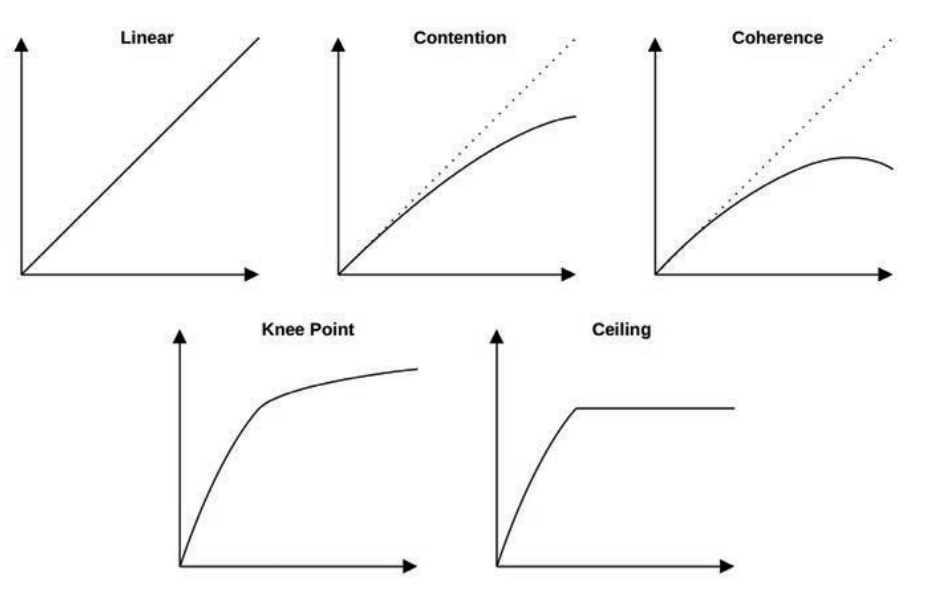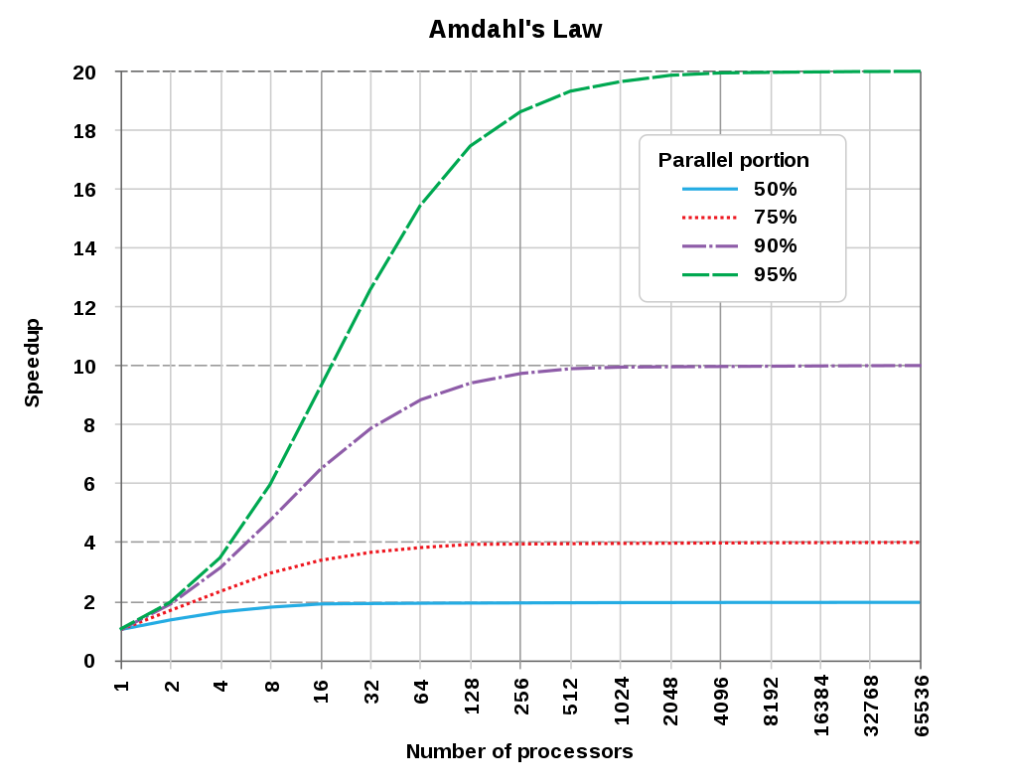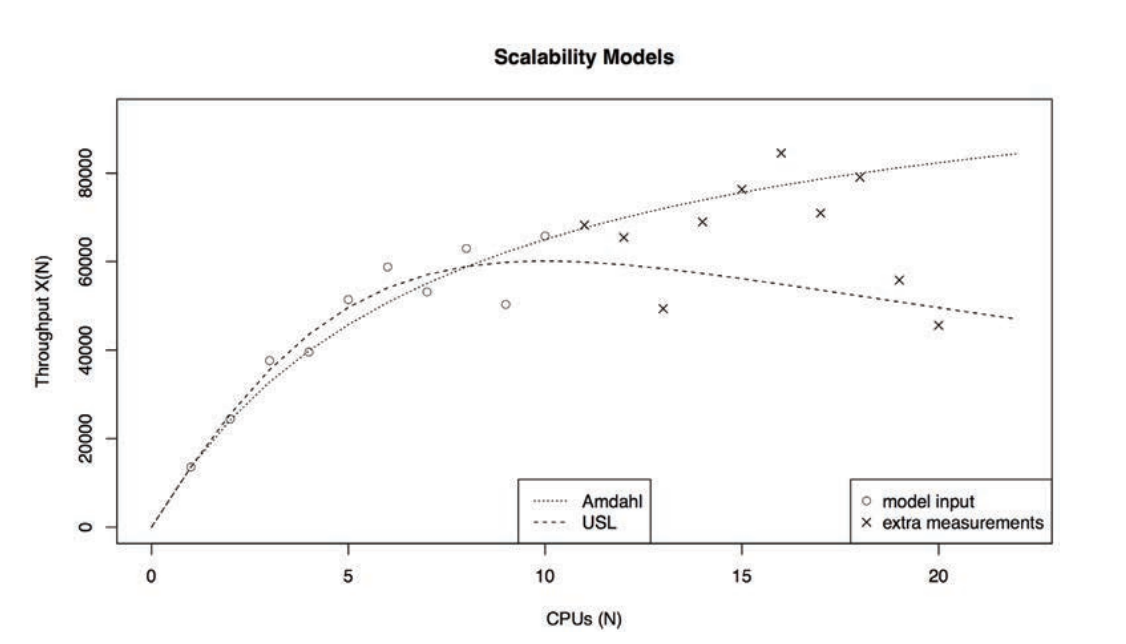# Amdahl 定律Ambdahl's law (安姆达定律，或安达尔定律)，是性能优化、横向扩展的理论依据之一。

## 1 Amdahl 定律公式

Amdahl 定于描述性能优化的极限。我们定义性能优化程度 S (Speedup) 为优化后性能与优化前性能的比值：

$S = \frac{Performance\ using\ the\ enhancement}{Performance\ not\ using\ the\ enhancemanet}$

$S = \frac{Execution\ Time\ without\ using\ the\ enhancement}{Execution\ Time\ using\ the\ enhancemanet}$

Amdahl 定律把一个系统分为两个部分：可优化的部分 $$p$$ ，和不可优化的部分 $$1-p$$ 。(这里 "可优化" 意思是使用这个技术可以提升性能的那部分；" 不可优化" 意思是这次优化使用的技术不能让这部分有性能提升。)

$E_{new} = E_{old} \times \left( (1-p) + \frac{p}{s} \right)$

$$E_{new}$$ 是优化后执行一个任务的时长；$$E_{old}$$ 是优化前一个任务消耗的时间；$$p$$ 是可优化的部分在整个系统中的百分比； $$s$$ 是可被优化的部分这次性能提升多少。

$S_{overall} = \frac{E_{old}}{E_{new}} = \frac{1}{(1-p)+\frac{p}{s}}$## 2 实际应用

(1) 实例1：给服务器换CPU

$S_{overall} = \frac{1}{(1-p)+\frac{p}{s}}= \frac{1}{0.6+\frac{0.4}{10}}\approx 1.56$

(2) 实例2：选优化方案

$S_{a} = \frac{1}{(1-0.2)+\frac{0.2}{10}} = 1.22$

$S_{b} = \frac{1}{(1-0.5)+\frac{0.5}{1.6}} = 1.23$

(3) 实例3：多线程

$S_{3} = \frac{1}{(1-0.8)+\frac{0.8}{4}}=2.5$

(4) 实例4：重构

$S_{4} = \frac{1}{(1-0.9)+\frac{0.9}{4}} \approx 3.08$

(5) 实例4: 换多核 CPU

$S_{5} = \frac{1}{(1-0.9)+\frac{0.9}{6}} = 3$

## 3 Amdahl 公式其他形式

$S=\frac{1}{(1-p)\frac{p}{s}}=\frac{s}{s(1-p)+p}=\frac{s}{1+(s-1)f}$

$C(N) = \frac{N}{1+\alpha(N-1)}$

## 4 Amdahl 定律的局限

Amdahl 定律是个理论值，在横向扩展问题里，它假设加一个服务器，执行某个部分的任务的速度就会增加多少倍，忽略了沟通的成本。服务器越多，网络通信也将会也多，增加的协调成本也会更高。这部分消耗被 Amdahl 定律忽略了。

$C(N) = \frac{N}{1+\alpha (N-1)+\beta N (N-1)}$